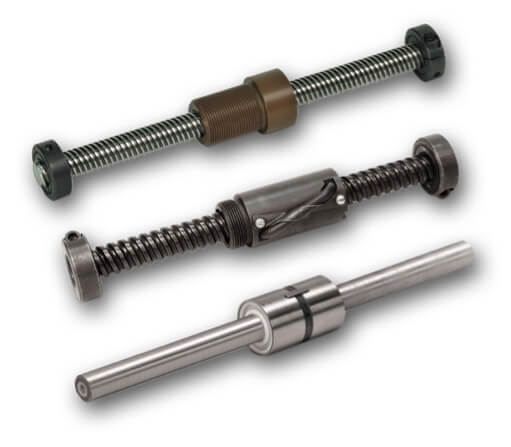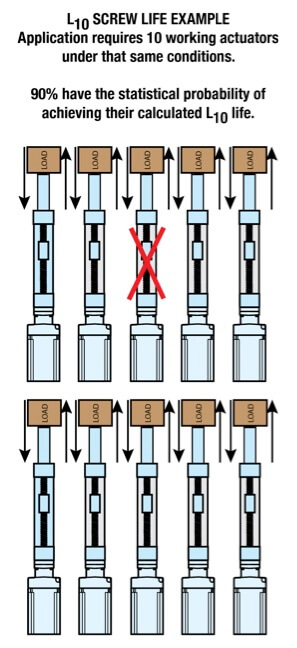# Failure not an option? How to avoid premature linear screw actuator failure

By Tolomatic on June 11, 2019Some components are more mission-critical than others.  A main worry of machine design engineers is making equipment that will last and avoiding need for field service calls to diagnose issues and replace failing components.  At their core, electric linear screw actuators deploy mechanical technology such as ball bearings, ball screws and roller screws that have a finite life.  These components do not last forever, even though that is the expectation of some customers.  When asked “How long will this actuator last?” we provide our customers with a way to calculate, estimate and size the electric linear screw actuator to achieve the desired life for their applications.

Every reputable actuator manufacturer should be able to provide the dynamic load rating (DLR) for their actuators.  If they cannot, our recommendation is to call Tolomatic.  Seriously, if a manufacturer cannot provide this, find another supplier and actuator solution.  Without a DLR specification, the life of the device cannot be estimated.  Estimating the life of a linear electric actuator is actually straight-forward for ball screw and roller screw actuators using the L10 life formula for ball bearings.  The life calculation focuses on these rolling elements because the screw/nut combination is an actuator’s critical moving component. We walk through the estimation process in our new guide, “Estimating life of a linear screw actuator designed with ball screw or roller screw.”

## Definitions of Critical Terms

Let’s start with definitions of critical terms you’ll need to know.Dynamic Load Rating (DLR): This is a number that represents a constant load under which a ball bearing device will achieve 1,000,000 revolutions (rotations) of rated life. It is usually provided by the manufacturer and represented by the letter C.

Constant load:  This is a load that remains unchanged along the full working cycle.

Equivalent Dynamic Load:  When an application has a varying load, you have to calculate the equivalent dynamic load or Pe. Equivalent dynamic load is a dynamic load acting on the screw which, if applied constantly, would have the same effect on screw life as the combined actual loads. In the case of constant load, equivalent load = actual load.

L10 (or B10) Life: L10 is a calculation of life at which 10% of bearings in the same application can be expected to fail due to classic fatigue failure. L10 life for a group of identical screw actuators operating under the same conditions is the number of revolutions (or distance of travel) which 90% of these actuators have the statistical probability to achieve.

This calculation provides a theoretical life estimate based on a collection of statistics. This is not a guarantee of performance but a guide for expected life.

## How to Calculate L10 Life with a Constant LoadTo estimate life with a constant load, the underlying formula is:

L10 = (C/Pe)3 x l

= Dynamic load rating (lbf) or (N)
Pe = Equivalent load (lbf) or (N
l = Screw lead (in/rev) or (mm/rev)

For example, in a case where:
C = 10,000 lbf
Pe = 5,000 lbf
l = 5 mm

L10 = (10,000/5,000)3 x 5 =
(2)3 x 5 =

8 x 5=
40 =
40 million mm

## How to Calculate L10 Life with a Varying Load

When a load varies during the working cycle you first have to calculate the equivalent dynamic load (Pe). Here’s the formula:

Pe = 3 √{ [L1(P1)3+L2(P2 )3+L3(P)3+Ln(Pn )3]/L}

Pe = Equivalent load (lbs) or (N)
Pn = Each increment at different load (lbs) or (N)
= Total distance traveled per cycle (extend + retract stroke) (L = L1 + L2 + L3 + Ln)
Ln = Each increment of stroke (in) or (mm) at different load
Then you can use the calculated Pe in the life calculation formula:

L10 = (C/Pe)3 x l

## How to Estimate Actuator Life in Time (Days, Years)

Here’s what you need to know for this calculation:

L10 = Life calculation in distance
L = Total distance traveled per cycle (extend + retract stroke)
CpM = Number of cycles per minute
HpD = Number of hours operated per day
DpY = Number of days of operation per year

Put that information into one of these formulas:

Life Estimate in Years =
(L10 / L) / [(CpM) X 60 min/hr X (HpD) X (DpY)]

Life Estimate in Days =
(L10 / L) / [(CpM) X 60 min/hr X (HpD)]

This gives you the estimated life of the ball screw or roller screw actuator in units of time.